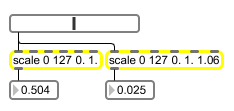# scale

Map an input range of values to an output range

## Description

scale maps an input range of float or integer values to an output range. The ranges can be specified with hi and lo reversed for invert-mapping. If specified, the mapping can also be exponential.

## Arguments

Name Type Opt Description
minimum-input maximum-input minimum-output maximum-output exponential-base int or float opt The first argument is the minimum input value, the second argument is the maximum input value. The third and fourth arguments are the minimum and maximum output values, respectively. An optional fifth argument specifies the nature of the scaling curve. The number is converted according to the following expression

y = b e-a log c ex log c

where x is the input, y is the output, a, b, and c are the three typed-in arguments, and e is the base of the natural logarithm (approximately 2.718282). The third argument must be a floating-point number greater than 1. The larger the value, the more steeply exponential the curve is. An appropriate value for this argument is 1.06. All five values can be changed via the object's five inlets. If only four arguments are provided and all four are of type int, scale will output integer values.

## Messages

 bang In left inlet: Performs the scaling operation on the most recently received int or float input value (i.e. if a list was the message thing received, the scale object will output the last item in the list). If the scaling ranges have changed since the previous input in the left inlet, the new ranges will be used for the scaling. int input [int] Converted to float. float input [float] In left inlet: The incoming value is scaled according to the mapping provided by the arguments, or values received in the other inlets. In second inlet: Sets the low input value. In third inlet: Sets the high input value. In fourth inlet: Sets the low output value. list input [list] In left inlet: A list of numbers sent to the left inlet will trigger the output of a list consisting of the results of performing the specified scaling operation on each item in the input list.

## Output

float: When scale receives a value in its leftmost inlet, that value is scaled to the indicated output range of values.
int: If only four arguments are provided and all four are of type int, scale will output scaled values as integers. Otherwise output is floating-point.

## ExamplesAn example of how to scale an integer slider into a useful range of floating-point values